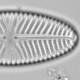## Introducing ggpalaeo

I’ve put some code I used for plotting figures for my soon-to-be-resubmitted manuscript into a package because I thought it might be useful to others.

The main use of `ggpalaeo` is to make `ggplot2` plots of transfer function diagnostics from the `analogue` and `rioja` packages.It will plot distances to nearest analogues and goodness-of-fit statistics by time or depth, and also plot transfer function performance plots. The diagnostic plots are perhaps better suited to supplementary material than the main text of a manuscript, but they are very pretty.

The other thing `ggpalaeo` does is make a couple of additions to `strat.plot` in `rioja`, allowing secondary axes (for example age in addition to depth) and summary plots (for example percent planktonic and benthic diatoms). Have a look at the examples.

You can install  `ggpalaeo` with

```devtools::install_github("richardjtelford/ggpalaeo")
```

You might need to install `devtools` first. Please let me know if you have any problems or suggestions for additions or improvements.## About richard telford

Ecologist with interests in quantitative methods and palaeoenvironments
This entry was posted in R, transfer function and tagged . Bookmark the permalink.

### 3 Responses to Introducing ggpalaeo

1.Dai says:

Really good bit of code to make using R and rioja even easier/better!

Is there a way to plot both a summary plot and a secondary axis in the same diagram? Tried playing with the par() arguments so they slot in to the diagram slightly differently, but no luck.

Cheers,

Dai

•richard telford says:

I’ve just pushed an update so that the par(“fig”) is automatically fixed.

2.richard telford says:

It is, but needs a little help.

```strat.plot(fos, yvar = chron\$Depth, y.rev = TRUE, xLeft = .22, xRight = 0.8, scale.percent = TRUE)
secondary.scale(yvar = chron\$Depth, yvar2 = chron\$Age, n = 5, y.rev = TRUE, ylabel2 = "Years")
par(fig = c(0, 1, 0, 1))
summary_plot(g = g, yvar = chron\$Depth, y.rev = TRUE, xLeft = 0.8)
```